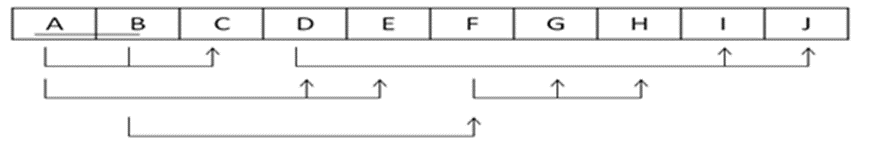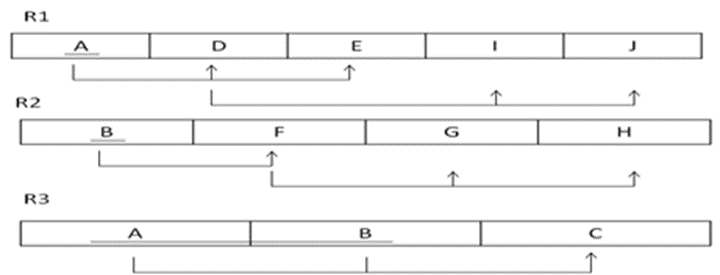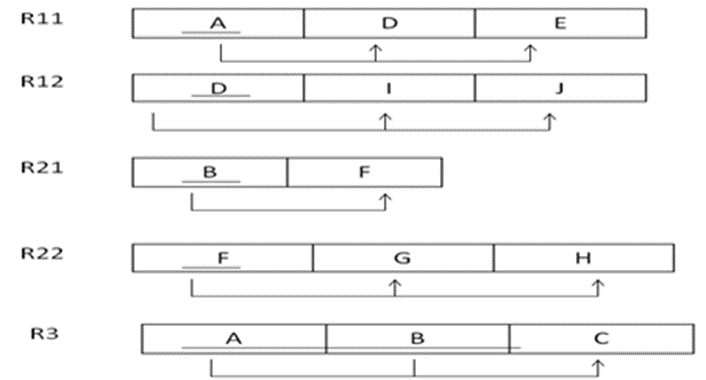Question:
Published on: 25 March, 2023

Consider the relation R={A,B,C,D,E,F,G,H,I,J} and the set of functional dependencies: F={AB → C, A → DE, B → F, F → GH, D → IJ}. Decompose R into 3NF.

A → DE, so AB → BDE (Augmentation)

AB → C, so AB → ABC (Reflexivity)

B → F and F → GH, so B → GH (Transitivity)

B → F and B → GH, so B → FGH (union)

B → FGH, so AB → ABFGH (Augmentation)

D → IJ, so DE → EIJ (Augmentation)

A → DE and DE → EIJ, so A → EIJ (Transitivity)

A → EIJ, so AB → ABEIJ (Augmentation)

AB → BDE, AB → ABC, AB → ABFGH, AB → ABEIJ, so,   AB → ABCDEFGHIJ (Union)

So, {A,B}+={A,B,C,D,E,F,G,H,I,J}.

So, AB is a candidate key.

Assume that, R is already in 1NF. Now to convert R into 2NF, we have to remove the partial dependency, if exists.We can notice that there are 2 partial dependencies exist. A → DE and B → F. So if we remove this, the Relation will be,Now, to convert these Tables into 3NF, we have to remove the transitive dependency, if exists. We can notice that, in R1, and R2 there are transitive dependency. SO, after remove all transitive dependency, the Relation will be,Random questions